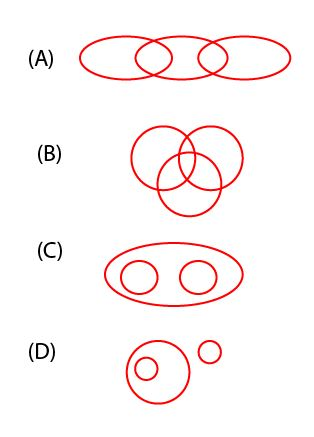• Save

# Important IBPS PO Questions with Answers

Vikram SinghLast year 3.6K ViewsEvery candidate works hard to join one of the reputed banks in the public sector banks of the country and succeed in popular exams like IBPS PO. As we all know that the level of competition in IBPS PO exam is very tough. Due to which it is also necessary to have knowledge of important questions asked in the exam.

It often happens that important questions from previous competitive exams are repeated again in the upcoming examinations. Therefore, most students discover the latest and important questions for practice as they have a chance to face the same questions in the competitive exam. So here I am sharing with you the important IBPS PO Question Answers from which you can easily practice and get help in increasing your score. So start your practice -

## Maths and Reasoning Related Questions with Answers

Q :

Two numbers are in the ratio of 5:6 and if 4 is added to the first number and 4 is subtracted from the second number then the ratio becomes 3:2. Find the difference between two numbers.

(A) 2.5

(B) 3.5

(C) 4.5

(D) 6.5

(E) None of these

Q :

Peter bought an item at 20% discount on its original price. He sold it with 40% increase on the price he bought it. The new sale price is greater than the original price (in percent) by:

(A) 10

(B) 8

(C) 7.5

(D) 12

Q :

A train crosses a platform in 30 seconds travelling with a speed of 60 km / h. If the length of the train be 200 metres, then the length ( in metres ) of the platform is

(A) 400

(B) 300

(C) 200

(D) 500

Q :

A scientist mixes 10% water in his solution but he is not content with it so he again mixes 10% more water in the previous mixture. What is the profit percentage of the scientist if he sells it at cost price:

(A) 15%

(B) 21%

(C) 18%

(D) 16%

(E) None of these

Q :

If the difference of the compound interest and the simple interest on a sum of money for 3 years is Rs. 186. Find the sum of money, if the rate of interest in both cases be 10 %

(A) 5500

(B) 7200

(C) 6500

(D) 6000

Q :

If Q is the brother of K. S is sister of K, and T is the sister of S, so let's say K, what is the relation of T?

(A) Brother

(B) Sister

(C) Brother - in - law

(D) Cannot be determined

Q :

A Person going to market for shopping and start walking from home 12 km east and reaches point M. From point M he takes left turn and walks 4 km and then he takes right turn and walked 6 km and again he takes right turn and walks 7 km and again takes right turn and reaches point N. If it is given that point M is in north from point N, then what is the distance between M and N?

(A) 7 km

(B) 6 km

(C) 5 km

(D) 4 km

(E) 3 km

Q :

Which of the following diagram indicates the best relation between Spinach, Brinjal, and Vegetables?(A) A

(B) B

(C) C

(D) D

Q :

In a certain code language, '-' represents 'x', '÷' represents '+', '+' represents '÷' and 'x' represents '-'. Find out the answer to the following question.
15 - 6 + 10 x 3 ÷ 2 = ?

(A) 24

(B) 9

(C) 2

(D) 8

Q :

If “S” denotes “multiplied by”, “V” denotes “subtracted from”, “M” denotes “added to” and “L” denotes “divided by”, then 8 V 10 M 96 L 6 S 9 = ?

(A) 140

(B) 142

(C) 134

(D) 144